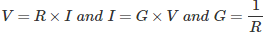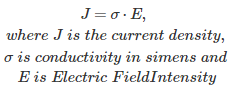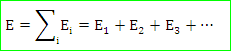# MCQs on Electromagnetic Fields

##### Page 1 of 20. Go to page 1 2 3 4 5 6 7 8 9 10 11 12 13 14 15 16 17 18 19 20
01․ Two parallel plates are separated by a distance D charged by V volt. The field intensity E is given by,
V × D
V / D
V × D²
V² / D

Electric field intensity between two points is always expressed as the potential difference between these two points divided by the distance between them. The strength of electric field or electric field intensity is measured by volt per meter or newton per meter.

02․ The right hand rule for determining the direction of the induced EMF was introduced by
Lenz
Fleming
Maxwell

The right hand rule for determining the direction of induced EMF was introduced by Fleming and it is well known as Fleming’s right hand rule. It is used to find the direction of induced emf of a generator.

03․ Whenever the magnetic flux changes with respect to an electric conductor or a coil, an EMF is induced in the conductor is Faraday’s
first law
second law
third law
fourth law

Faraday’s law of electromagnetic induction states
1. Whenever a changing flux links with a conductor, there will be an EMF induced in the conductor.
2. The magnitude of this induced EMF is directly proportional to the rate of change of flux linkage with respect to time.
These two statements are well-known as Faraday’s first and second law of electromagnetic induction respectively.

04․ Which of the following represents ohms law
V = RI
J = σE
I = GV
All of the above

These are the different forms to represent the ohms law.
• Ohms law states that at constant temperature potential difference across the element is directly proportional to current flowing through the element.• Ohms law states that at constant temperature current density is directly proportional to field intensity.• Ohms law is valid only when temperature and conductivity of the material is constant.

05․ Conductor is static and the field is varying then emf will be induced. This principle is called
virtually induced emf.
dynamically induced emf.
static induced emf.
none of these

According to the Faraday's law of electromagnetic induction principle, the emf will be induced whenever conductor cuts a varying field or vice versa. The emf will be induced when an alternating magnetic field cuts the rotor conductor.

06․ As a result of reflection from a plane conducting wall, electromagnetic waves acquire an apparent velocity greater than the velocity of light in space. This is called the
velocity of propagation.
normal velocity.
group velocity.
phase velocity.

The phase velocity of a wave is the rate at which the phase of the wave propagates in space. This is the speed at which the phase of any one frequency component of the wave travels. Basically, the speed of this velocity is greater than the velocity of light in space.

07․ Two infinite parallel metal plates are charged with equal surface charge density of the same polarity. The electric field in the gap between the plates is
same as that produced by one plate.
double the field produced by one plate.
dependent on coordinates of field points.
zero.

The equal surface charge densities are having same charge either positive or negative. So, same charge repels each other. Hence, the resultant electric field in the gap between the plates is zero.

08․ Inside a hollow conducting sphere
electric field is zero.
electric field is a non zero constant.
electric field changes with magnitude of the charge given to the conductor.
electric field changes with distance from the center of the sphere.

For any conducting sphere, the charge enclosed is zero and hence, the electric field inside it is zero. This is an application of the Gauss Law. In fact Gaussian surface includes no charges inside it.

09․ Electric field intensity (E) at ant point in an electric field is equal to
The total electric field at any point is equal to the vector sum of the separate electric fields that each point charge would create in the absence of the others. That is,The electric field is nothing but the potential gradient of that particular point.